# On the epoch of the Antikythera mechanism and its eclipse predictor

## Abstract

The eclipse predictor (or Saros dial) of the Antikythera mechanism provides a wealth of astronomical information and offers practically the only possibility for a close astronomical dating of the mechanism. We apply a series of constraints, in a sort of sieve of Eratosthenes, to sequentially eliminate possibilities for the epoch date. We find that the solar eclipse of month 13 of the Saros dial almost certainly belongs to solar Saros series 44. And the eclipse predictor would work best if the full Moon of month 1 of the Saros dial corresponds to May 12, 205 BCE, with the exeligmos dial set at 0. We also examine some possibilities for the theory that underlies the eclipse times on the Saros dial and find that a Babylonian-style arithmetical scheme employing an equation of center and daily velocities would match the inscribed times of day quite well. Indeed, an arithmetic scheme for the eclipse times matches the evidence somewhat better than does a trigonometric model.

This is a preview of subscription content, log in to check access.

1. 1.

The Babylonians had no way to treat the lunar parallax. Some writers characterize also the lunar eclipses in a Saros scheme as “eclipse possibilities.” The advantage of this usage is that it acknowledges that the Babylonian astronomers knew that sometimes a predicted lunar eclipse does not occur or that sometimes one occurs in the wrong month. Still, the prediction of lunar eclipses was pretty accurate, so to describe these predictions as giving mere possibilities seems an unduly positivistic characterization of what the Babylonians were up to.

2. 2.

Original publication of the eclipse glyphs is then known: (Freeth et al. 2008). These (both the journal article and the online Supplementary Notes) contained some errors in the presentation of the eclipse data. These have been corrected in a new version of the Supplementary Notes posted at http://www.nature.com/nature/journal/v454/n7204/extref/nature07130-s1.pdf, to which readers should now refer. (Most of these errors were corrected by Beatriz Bandeira of the Universidad Nacional Tres de Febrero of Argentina). We have taken the times of the eclipses from the most recent analysis made by Alexander Jones in a still unpublished paper. They differ in some cases from the times published in the Supplementary Notes of Freeth et al. (2008). The following differences should be noted. We list first the eclipse cell and eclipse type, then the reading of Freeth et al., and finally Jones’s reading. 13S: day 1 or 4, day 1. 78S: night 1, day 1. 120M: day 8, day 6. 172M: no reading, night 6. 172S: no reading, day 12. 178S: most unclear, day 9. In all cases, we followed Jones’s readings. We excluded from the time analysis three eclipses: 120M because Jones’s reading is uncertain and 67S and 72H because the reading is incomplete. For S119 both Jones and Feeth et al. 2008 read $$\iota$$[ ] which could be 10, 11 or 12, so we assume the mean value, 11. A lunar eclipse in month 61 has recently been discovered (Anastasiou et al. 2014). Our eclipse analysis was completed before this discovery was made; but the new glyph fit right into the already reconstructed Babylonian pattern with no changes required.

3. 3.

This is also discussed in Aaboe et al. (1991, p. 17).

4. 4.

That is, one modifies the solar Saros scheme without modifying the lunar scheme, in such a way that the solar scheme that began 4 EP earlier than the lunar one now begins 4 EP later than the lunar one. This could sound odd if one supposes that the solar schemes originated as modifications of the lunar schemes. Why would you recalibrate the solar and not the lunar if the solar is based on the lunar? But it seems that in the case of re-calibration the dependence is in the other direction. Steele (2000a, p. 443) suggests that one particular lunar recalibration, in $$-$$110, was unnecessary but nevertheless took place to coincide with a solar re-calibration. He also suggests that “Since solar eclipses can occur at greater nodal elongations than lunar eclipses, it was necessary to revise the solar Saros scheme more often than the lunar Saros.”

5. 5.

For example, Steele (2000a, p. 442) points out that the circumstances of the $$-$$110 recalibration are not completely clear.

6. 6.

For the Moon’s argument of latitude (the mean angular distance of the Moon from its ascending node), see (Meeus 2009, p. 338, Eq. 47.5). In our calculations, we used an Excel program, based on Meeus’s formula, written by Dennis Duke.

7. 7.

The groupings of eclipses into 8-8-7-8-7s are from Steele (2009, Table 4). The Saros series numbers for these eclipses come from Espenak (Five Millennium Catalog of Solar Eclipses).

8. 8.

However, the Britton rule could have been compatible with Babylonian practice before about $$-$$260: As we have seen, during the earlier period, while a solar 8-8- began with the Moon at D for the solar eclipse, the lunar 8-8- also began with the Moon at D for the lunar eclipse.

9. 9.

Cells 19 and 7 are, at least in theory, extant in fragments F and A, respectively. Cell 7 is almost impossible to see. It is also impossible to see Cell 8, or at least to read any letter. But cell 19 is clearer, and it seems reasonably clear that there is nothing there. While we cannot be sure from direct examination of these cells, the sequence of index letters is enough to decide the issue.

10. 10.

For example, the solar recalibrations that took place in $$-$$387 and in $$-$$265.

11. 11.

We take the year to be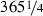days and assume the Metonic relation 235 synodic months $$=$$ 19 years.

12. 12.

The maximum value of the Moon’s equation of center is actually about $$6.3^{\circ }$$; but we are concerned with eclipses, i.e., with phenomena taking place at new and full Moon. In these situations, the equation of center is reduced by the evection by about $$1.3^{\circ }$$; thus, the effective maximum equation of center at new and full Moons is about $$5^{\circ }$$. We neglect higher order terms in the equation of center, the annual equation, and other small perturbations of the Moon’s longitude.

13. 13.

By the instant of opposition, we mean instant when the Moon’s mean longitude is equal to the Sun’s mean longitude $$+$$ $$180^{\circ }$$. $$\bar{h} _0$$ is the local time of day at which this happened. The time of true opposition would be $${h}_{0}$$, which differs from $$\bar{h} _0$$ due to the contributions of the lunar and solar equations.

14. 14.

For an introduction to the $$F$$-test, see (Ramsey and Schafer 2002, pp. 122–127, 280–285), with tables at pp. 720–727. A more mathematically detailed explanation is given in Fox (2008, pp. 200–202). Functions for calculating the p value from the F-statistic are provided in Excel and other commonly available programs.

15. 15.

For the middle of the eclipse, here, more precisely, is what we used: For eclipses that actually occurred, we used the time of greatest eclipse from the Espenak solar eclipse catalog. For eclipses predicted by the Babylonians which did not really occur, we use the time of true conjunction, from (Espenak, Six Millennium Catalog of Phases of the Moon).

16. 16.

Espenak actually gives the time of greatest eclipse in terms of TD. We have converted to UT.

17. 17.

Using all 22 eclipses, we get for the mean timing error $$-$$0.73 h.

18. 18.

But in the analysis in this paper, we use the length of the anomalistic month that is implicit in the Antikythera mechanism, which is (223/239) times the synodic month. For the synodic month, we use $$365.25^{\mathrm{d}} (19/235)$$. This makes no practical difference: An anomalistic month of 27.556 days for System B and Geminus, 27.554 days implicit in the AM.

19. 19.

Our technique is the same as that used in Sect. 13. We compute the lunar and solar equations of center for the given models and assume that these need to be compensated for by the Moon and Sun running at their mean relative motion of $$12.2^{\circ }/\hbox {day}$$. Once we know the locations of the perigees, it is possible to apply a more sophisticated reckoning, in which we take into account the variable speeds of the Moon and Sun. This is done in Sect. 19.

20. 20.

The mean anomaly does not have a clear-cut definition as an angle in this non-geometrical model. The parameter determined from the fit is the offset of the zero crossing from $$t = 0$$ in Fig. 22, which is 0.01392 of an anomalistic period. Note that at $$t = 0$$, the eclipses are slightly late and getting later; thus, the equation of center is slightly negative and getting more negative, i.e., we are near (and just after) apogee, by $$0.01392 \times 360^{\circ } = 5.0^{\circ }$$, hence the value $$185.0^{\circ }$$.

21. 21.

The mean anomaly does not have a clear-cut definition as an angle in this non-geometrical model. The parameter determined directly from the fit is the t-value of the most negative point in Fig. 23, which is 0.24084 of a year. The zero crossings of the graph are the moments when the equation of center is zero, which we associate with “perigee” and “apogee.” As can be seen, at $$t = 0$$, the eclipses are very slightly early, and getting earlier. Hence, we are near, and just after, apogee. The zero crossing would have occurred at $${t} = -0.01028$$ year. Hence, we take the mean anomaly to be $$0.01028 \times 360^{\circ }$$ beyond apogee, i.e., $$183.7^{\circ }$$. Note that in this Babylonian-inspired model, the maximum and minima are not uniformly spaced. However, the zero crossings are.

22. 22.

The Babylonian-style method described here is actually a pretty good predictor of eclipse times: Its RMS discrepancy with the true eclipse times is 1.51 h.

23. 23.

The maximum values of the equations of center corresponding to these epicycle radii are about $$1.75^{\circ }$$ for the Sun and $$4.90^{\circ }$$ for the Moon.

24. 24.

Recall that $$\bar{\lambda } =\bar{\alpha }+ \Pi$$, where for the Sun we may put $$\Pi = 65.5^{\circ } - 180^{\circ }$$. Thus, $$\bar{\lambda } =\bar{\alpha }- 114.5^{\circ }$$. For the arbitrary value of the solar mean anomaly $$\bar{\alpha }$$, put in any of the initial values of the mean solar anomaly $$\varphi _{\odot }$$ from column 5 of Table 12. For example, if we use $$\varphi _{\odot }= 175.5^{\circ }$$, we find $$\bar{\lambda }= 61.0^{\circ }$$ for the mean longitude of the Sun at the opposition of month 1.

## References

1. Aaboe, A., J.P. Britton, J.A. Henderson, O. Neugebauer, and A.J. Sachs. 1991. Saros cycle dates and related Babylonian astronomical texts. Transactions of the American Philosophical Society, New Series 81(6): 1–75.

2. Anastasiou, M., J.H. Seiradakis, C.C. Carman, and K. Efstathiou. 2014. The Antikythera mechanism: The construction of the Metonic pointer and the back plate dials. Journal for the History of Astronomy 45:1–26.

3. Bickerman, E.J. 1980. Chronology of the ancient world. Ithaca: Cornell University Press.

4. Britton, John P. 1989. An early function for eclipse magnitudes in Babylonian astronomy. Centaurus 32: 1–52.

5. Espenak, Fred. Eclipses and the Saros. http://eclipse.gsfc.nasa.gov/SEsaros/SEsaros.html.

6. Espenak, Fred. NASA Eclipse Web Site. NASA Five-Millennium Catalog of Lunar Eclipses. http://eclipse.gsfc.nasa.gov/LEcat5/LE-0099-0000.html.

7. Espenak, Fred. NASA Eclipse Web Site. Five-Millennium Catalog of Solar Eclipses. http://eclipse.gsfc.nasa.gov/SEcat5/SEcatalog.html.

8. Espenak, Fred. NASA Eclipse Web Site. Javascript Solar Eclipse Explorer. http://eclipse.gsfc.nasa.gov/JSEX/JSEX-index.html.

9. Espenak, Fred. NASA Eclipse Web Site. Six Millennium Catalog of Phases of the Moon. http://eclipse.gsfc.nasa.gov/phase/phasecat.html.

10. Evans, James, and Christián Carlos Carman. 2013. On the epoch of the Antikythera Mechanism (PowerPoint presentation). http://www.lorentzcenter.nl/lc/web/2013/570/presentations/index.php3?wsid=570&type=presentations&venue=Oort.

11. Evans, James, and Christián Carlos Carman. 2014. Mechanical astronomy: A route to the ancient discovery of epicycles and eccentrics. In From Alexandria, through Baghdad: Surveys and studies in the ancient Greek and medieval Islamic mathematical sciences in honor of J. L. Berggren, ed. Nathan Sidoli, and Glen Van Brummelen, 145–174. heidelberg: springer.

12. Evans, James, Christián Carlos Carman, and Alan S. Thorndike. 2010. Solar anomaly and planetary displays in the Antikythera Mechanism. Journal for the History of Astronomy 41: 1–39.

13. Evans, James, and J. Lennart Berggren. 2006. Geminos’s introduction to the phenomena: A translation and study of a Hellenistic survey of astronomy. Princeton: Princeton University Press.

14. Fox, John. 2008. Applied regression analysis and generalized linear models. Los Angeles: Sage.

15. Freeth, Tony. 2014. Eclipse prediction on the Antikythera Mechanism. PLOS One (Public Library of Science). http://dx.plos.org/10.1371/journal.pone.0103275.

16. Freeth, Tony, et al. 2006. Decoding the ancient Greek astronomical calculator known as the Antikythera Mechanism. Nature 444. doi:10.1038/nature05357. Supplementary Information is linked to the online version of the paper at www.nature.com/nature.

17. Freeth, Tony, Alexander Jones, John M. Steele, and Yanis Bitsakis. 2008. Calendars with Olympiad display and eclipse prediction on the Antikythera Mechanism. Nature 454. doi:10.1038/nature07130. Additional information is available in the “Supplementary Notes” at www.nature.com/nature.

18. Iversen, Paul. 2013. http://apaclassics.org/annual-meeting/151iversen.

19. Meeus, Jean. 2009. Astronomical algorithms, 2nd ed. Richmond: Willmann-Bell.

20. Morgan, John D. 2013. The season of Karneios. (A presentation at the conference The Antikythera Mechanism: Science and innovation in the ancient world, held in Leiden). A PDF of the presentation is posted at the conference web site. http://www.lorentzcenter.nl/lc/web/2013/570/presentations/index.php3?wsid=570&type=presentations&venue=Oort.

21. Neugebauer, O. 1975. A history of ancient mathematical astronomy. Berlin, Heidelberg, New York: Springer.

22. Ramsey, Fred, and Daniel Schafer. 2002. The statistical sleuth: A course in methods of data analysis, 2nd ed. Belmont, CA: Brooks/Cole, Cengage Learning.

23. Price, Derek de Solla. 1974. Gears from the Greeks: The Antikythera mechanism—A calendar computer from ca. 80 B.C. Transactions of the American Philosophical Society, n.s., lxiv/7.

24. Smart, W.M. 1977. Textbook on spherical astronomy, 6th ed. Cambridge: Cambridge University Press.

25. Steele, John M. 2000a. Eclipse prediction in Mesopotamia. Archive for History of Exact Sciences 54: 421–454.

26. Steele, John M. 2000b. Observations and predictions of eclipse times by early astronomers. Dordrecht, Boston: Kluwer Academic Press.

27. Toomer, G.J. 1984. Ptolemy’s Almagest. London: Duckworth.

## Acknowledgments

We are grateful to James Bernhard for a discussion of statistical issues. Alexander Jones generously shared preliminary results of his own unpublished research which disclosed the existence of a lunar equation of center embedded in the Antikythera mechanism eclipse times. This turned out to be crucial for our project—it is the lunar anomaly that allows one to unambiguously identify a particular solar Saros series. Dennis Duke gave valuable help in calculation and programming. We thank John Steele for answering our questions about the Babylonian Saros schemes. We thank Paul Iversen and John Morgan for their lively and helpful discussion of previous versions of the paper. The Antikythera Mechanism Research Project has, as always, been extremely generous in sharing images and data. James Evans expresses his thanks to the University of Puget Sound for research funds that helped make this work possible. Christián Carman would like to express his thanks for the support of Research Project PICT-2010-0319 of the Agencia Nacional de Promoción Científica y Tecnológica of Argentina. While we were in the last stages of completing our manuscript, an article by Tony Freeth was published that focuses on some of the same issues that we have studied (Freeth 2014), but it appeared too late for us to make any use of it. The work published here is independent of Dr. Freeth’s study.

## Author information

Authors

### Corresponding author

Correspondence to James Evans.

Some of the main results of this paper were presented in Leiden, on June 20, 2013, at the week-long conference, The Antikythera Mechanism: Science and Innovation in the Ancient World organized by Niels Bos, Mike Edmunds, Alexander Jones, Onno van Nijf, and Rien van de Weygaert. A PowerPoint of our presentation, “On the Epoch of the Antikythera Mechanism,” was posted on the conference Web site, available to participants (Evans and Carman 2013). A shorter version of the talk was presented at the International Congress of History of Science, Technology and Medicine, in Manchester, UK, on July 22, 2013, under the title “Approaches for the epoch of the Antikythera mechanism,” in Session S092-B.

Communicated by : A. Jones.

## Appendices

### Appendix 1: The possible distributions of lunar eclipses on the Saros dial

In their study of the lunar eclipses, Freeth et al. rightly remark that the critical factor in the analysis is where the 5-month intervals are. So, in their analysis, they fix one of the “7-” groups, identifying two 5-month intervals, one between months 79 and 84 and the other between 120 and 125. Then, they say,

Therefore any Babylonian scheme that is consistent with the glyphs must have 5-month [intervals] between Months 79 and 84 and between months 120 and 125. This fixes one of the ‘7’s in the 8-7-8-7-8 pattern and it is easy to see that this then fixes the whole Babylonian pattern relative to the glyphs (Freeth et al. 2008, p. 32).

But it is not correct to suppose that knowing one of the “7-” groups is enough for fixing the whole pattern. In the Babylonian scheme, there are two different “7-” groups—one followed by two groups of 8 eclipses and the other followed by only one—so it is necessary to know which of the two “7-” groups has been identified. Thus, it turns out that there is not just one possible distribution of lunar eclipses that is consistent with the Babylonian scheme and with the inscriptions on the Antikythera mechanism, but rather two different distributions, which are in Table 1.

### Appendix 2: The possible distributions of solar eclipses

Solar EPs are inscribed in months 13, 25, 72, 78, 119, 125, 131, 137, 172, 178, and 184. As Freeth et al. noted, between cell 184 and cell 13 (carrying forward beyond the end of the 223-month dial into the beginning of the next Saros cycle), there must be two 4-month gaps. For between cell 184 and cell 13 are cells for 51 months, which means that we have room for an “8,” plus 2 4-month gaps (one before and one after the “8”). Alternatively, we could have a “7”, plus 2 4-month gaps (one before and one after the “7”), plus six extra months that would belong to the next or the previous group. Thus, the 2 4-month gaps could be separated either by an “8” or by a “7”.

Case i :

Suppose that the 2 4-month gaps are separated by an “8.” Then, the 4-month gaps must be at the extremes, i.e., one just before month 13 and the other just after month 184. So, the 2 4-month gaps consist of months 9–12 and 185–188. And there is an “8” from 189 through 8. All three solutions under Case i satisfy these conditions.

The Saros pattern takes the form 8-8-7-8-7- (or a cyclic permutation thereof). So we will have sub-cases, depending on which of the three possible positions is taken by the “8” that occupies cells 189 through 8. We must allow for the possibility that this “8” is:

• the second “8” of two successive “8”s (subcase 1)

• or the first “8” of two successive “8”s (subcase 2)

• or the lone “8” (subcase 3).

Suppose, alternatively, that the two 4-month gaps (between cell 184 and cell 13) are separated by a “7”. Then, we have two additional cases:

Case ii :

One of the 4-month gaps is just after month 184, and is followed by the “7”. Thus, there is a “7” from 189 through 2. Both solutions under Case ii satisfy this condition. This “7” is either:

• the “7” that follows two successive “8”s (subcase 4)

• or the “7” that follows a lone “8” (subcase 5).

Case iii :

One of the 4-month gaps is just before 13 and is preceded by the “7”. Thus, there is a “7” from 195 through 8. Both solutions under Case iii satisfy this condition. This “7” is either:

• the “7” that follows two successive “8”s (subcase 6)

• or the “7” that follows a lone “8” (subcase 7).

So, to sum up, there are seven solar patterns that would be consistent with the extant glyphs and with a Babylonian-style 8-8-7-8-7- distribution scheme and these are shown in Table 2.

### Appendix 3: Alternative determination of the A and D nodes for the solar eclipses

We mentioned above that in the Babylonian Saros record the first eclipse of an 8-8- group is consistently a D (an eclipse at the descending node). But there are no astronomical reasons for that. It is clearly a convention, because the first eclipse of an 8-8- group could just as well be an A. So, if we do not want simply to assume that the Saros dial of the Antikythera mechanism was based on the Babylonian records, we have to explore both possibilities. The sequence actually followed by Babylonian records, starting with a D eclipse at the beginning of the 8-8-, is represented in the left-most column of Table 9. The alternative sequence, starting with an A eclipse, can be obtained by exchanging A for D in the same column.

Now, we know that the solar eclipse in month 13 comes 11 months after a solar eclipse in month 2. (For all seven possible solar solutions require an eclipse in month 2). Only eclipses that are first or second in the 8- or 7- groups are 11 months after another EP. Thus, whether we start the 8-8- with a D or with an A, we will always have an $$\hbox {A}_{1}$$ and $$\hbox {D}_{1}$$ as the first two EP. (What will change is the order—either $$\hbox {D}_{1}$$ $$\hbox {A}_{1}$$ or $$\hbox {A}_{1}$$ D$$_{1})$$. Now, applying the omission rule, we know that $$\hbox {A}_{1}$$ eclipses must be omitted; but the eclipse in month 13 is an extant eclipse: Consequently, it is a $$\hbox {D}_{1}$$. Therefore, no matter which of the seven solar patterns were adopted for Saros dial, and no matter whether the 8-8- began with an A or a D, we can be sure that the eclipse in month 13 is a $$\hbox {D}_{1}$$.

### Appendix 4: Examples of 24-h correction analysis applied to the inscribed eclipse times

For example, consider eclipses 78S and 119S (solar eclipses in months 78 and 119):

Eclipse Change in lunar mean anomaly from eclipse 20M Recorded advance in time from eclipse 20M Advance in time from 20M expected due to mean motions Advance in time from 20M over and above effect of mean motions
78S $$251.03^{\circ }$$ $$7^{\mathrm{h}}$$ $$13.31^{\mathrm{h}}$$ $$-6.31^{\mathrm{h}}$$
119S 230.05 5 7.67 $$-$$2.67

By virtue of the number of anomalistic months elapsed from the opposition of month 20, we see that the Moon’s mean anomaly must have been similar in both eclipses—$$251^{\circ }$$ greater than the mean anomaly at the opposition of month 20 (in the case of eclipse 78S) and $$230^{\circ }$$ greater than the mean anomaly at the opposition of month 20 (in the case of eclipse 119S). Thus, the effect of the lunar anomaly on the time shift between these two eclipses was small. The inscribed glyphs show that the time of day of eclipse 78S advanced by 7 h from the time of day recorded for eclipse 20M (the lunar eclipse of month 20). The number of synodic months elapsed from 20M implies that the time of day for eclipse 78S should have shifted forward by $$13.31^{\mathrm{h}}$$ due to the mean motions alone. Thus, the advance in time over and above the effect of the mean motions was $$7 - 13.31 =\,{-}6.31^{\mathrm{h}}$$, for eclipse 78S. Similarly, the advance in time of eclipse 119S with respect to 20M, over and above the effect of the mean motions was $$-2.67^{\mathrm{h}}$$. The “signal” that we are trying to explain consists of the numbers in column (5)—the advance in time over and above the effect of the mean motions. Now, we have chosen two eclipses at which the lunar mean anomalies were similar; thus, the effect of the lunar anomaly on the signal is small. The two values in column (5) should rarely differ by more than 8 h, which is the maximum effect of the solar anomaly. The difference between $$-$$6.31 and $$-$$2.67 is $$<$$8 h, so we may be confident that no correction is required to the times in column (3).

But now consider eclipses 25S, 137S, 172M and 178S:

Eclipse Change in lunar mean anomaly from eclipse 20M Recorded advance in time from eclipse 20M Advance in time from 20M expected due to mean motions Advance in time from 20M over and above effect of mean motions
25S $$322.06^{\circ }$$ $$12^{\mathrm{h}}$$ $$10.07^{\mathrm{h}}$$ $$1.93^{\mathrm{h}}$$
137S 334.98 18 21.00 $$-$$3.00
172M 326.10 24 (or 0) 16.54 7.46 (or $$-$$16.54)
178S 313.99 15 15.36 $$-$$0.36

These have lunar mean anomalies that span a narrow range of about $$21^{\circ }$$. So it is clear that the difference in the glyph times between 20M and 172M must be treated as 24 h and not 0 h. If we took it as 0 h, then the values in column (5) for 137S and 172M would differ by over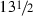h, which is out of the realm of possibility. Now, with column (3) for 172M chosen to be 24 h, it is true that the values in column (5) for 137S and 172M differ by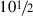h, a bit more than the 8-h maximum effect for the solar anomaly. But the lunar anomalies for these two eclipses differ by about $$9^{\circ }$$, so the maximum possible lunar effect on the time shift is about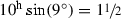h; thus a total lunar plus solar effect of up to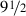h is conceivable, which is perhaps close enough to the attestedh difference. For, we should certainly allow for the possibility of some scattered error (which, in view of the uncertainties about the time conversion, discussed above, could easily amount to an hour or somewhat more). Thus, all four eclipses are in decent accord, but only if we put column (3) at 24 h for 172M.

Consider one last example involving the eclipses 137M and 178M:

Eclipse Change in lunar mean anomaly from eclipse 20M Recorded advance in time from eclipse 20M Advance in time from 20M expected due to mean motions Advance in time from 20M over and above effect of mean motions
137M $$142.06^{\circ }$$ $$11^{\mathrm{h}}$$ (or $$-$$13) $$2.63^{\mathrm{h}}$$ $$8.37^{\mathrm{h}}$$ (or $$-$$15.63)
178M $$121.08^{\circ }$$ 3 20.99 $$-$$17.99

Using the recorded advances in time with respect to $$20\hbox {M}\,(11^{\mathrm{h}}$$ for the first eclipse and $$3^{\mathrm{h}}$$ for the second) gives values in column (5) that differ by some 14.38 h. This far exceeds the 8-h maximum for the solar effect. Even when we add in a maximum possible lunar effect of $$10^{\mathrm{h}}\sin (21^{\circ }) = 3.58^{\mathrm{h}}$$, for the lunar anomalistic difference of $$21^{\circ }$$, we could only hope to explain a difference of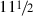h in column (5). Thus, it is clear that either 24 h needs to be subtracted for 137M in column (3), or 24 h added for 178M. Deciding which is required involves fitting these two eclipses into the larger pattern.

### Appendix 5: Identification of outliers

We suspect that, for several of the month cells, there is something wrong with the times of the eclipses inscribed on the Saros dial, perhaps a copying error or a calculation error.

Figure 31 displays the time by which each of 18 eclipses arrived early or late, by comparison with what would be expected from mean motions alone. The time is reckoned in synodic months from the opposition of month 1. So the basic signal we are trying to explain has an amplitude of some 10–15 h.

In Fig. 32, we have plotted the data of Fig. 31 minus the lunar equation of center function. The graph therefore displays the residuals left over after the lunar equation of center is taken into account. The tightening of the plot shows overwhelming evidence of the existence of a lunar equation of center function in the basic data.

In Fig. 33, we plot the data of Fig. 31 minus the sum of the lunar and solar equations of center. The further tightening of the residuals (between Figs. 3233) is evidence of the existence of the solar equation of center in the basic data. Figure 34 is the same as Fig. 33, except that the four eclipses excluded as outliers (13S, 120M, 125M, and 184M) have been added to the figure and are represented by the hollow squares. (The solar and lunar fits are still determined by the eclipses represented by the 18 diamonds). Eclipses 13S and 125M are so out of character with the rest of the data, that their exclusion seems quite justifiable. For 120M and 184M, the case is less clear-cut. We exclude them for having residuals $$>$$5 h. The exclusion of these four eclipses produces a considerable improvement in the quality of the lunar fit (Fig. 7 contrasted with Fig. 9), which is a stronger argument for their validity than the improvement produced in the solar fit (Fig. 8 compared with Fig. 10). However, we present our results both with and without the exclusion of these outliers.

Alexander Jones (personal communication) independently found that the times of eclipses 13S, 125M, and 184M are problematical. The identification of outliers depends to some extent on the method used. He also finds that 172M is problematical (while we found 120M). In Fig. 14, 172M is the point with largest positive value and seems unproblematical; and in Fig. 33, it is the point with the greatest positive value, but does not really seem to be an outlier. A key point of technique is that it is important to fit the solar and lunar equations of center simultaneously. One will not get the correct fits by fitting the lunar equation first and then fitting the solar equation to the residuals, since these two functions are not orthogonal.

### Appendix 6: The Babylonian node conventions and the Britton and Steele rules

As we have seen, the Babylonians put the first solar EP of an 8-8- consistently at the descending node D. And, after about $$-$$250, they put the lunar EP that starts the lunar 8-8- at the A node. We shall prove that this necessarily entails the Steele rule or the alternative Steele rule. On the other hand, if we keep the solar EP that starts the solar 8-8-7-8-7- at D, but also choose to place the lunar EP that starts the lunar 8-8-7-8-7- at D, this necessarily entails the Britton rule. Thus, the node conventions discussed in Sect. 6 are intimately linked with the Steele and Britton rules discussed in Sect. 5.

Let us reckon the solar eclipse pattern from the first eclipse in the solar 8-8-, at a moment we shall call time 0. And we shall measure time in synodic months (sm). The solar eclipse at the beginning of the 8-8- will of course be the most northerly $$\hbox {D}_{1}$$. We shall then prove that (a) if we want to start the lunar pattern from the most southerly $$\hbox {A}_{1}$$, we must necessarily follow Steele rule (or the alternative Steele rule). But, (b) if we want instead to start the lunar pattern from the most northerly $$\hbox {D}_{1}$$, then we must necessarily follow the Britton rule.

Proof of (a). An $$\hbox {A}_{1}$$ lunar eclipse falls 23.5 synodic months earlier or later than a $$\hbox {D}_{1}$$ solar eclipse. To see this, consider the $$\hbox {D}_{1}$$ solar eclipse at time 0. Then 4 solar EPs later, at time 24.0 sm, there will be another solar EP. There is a possibility of a lunar EP two weeks before this, at time 23.5 sm; for $$23.5\,\hbox { sm} \times (242\,\hbox {dm}/223\,\hbox {sm}) = 0.5022\,\hbox { dm}$$, over and above complete draconic months, or very nearly $$180^{\circ }$$ (actually about $$180.79^{\circ }$$). Therefore, the lunar EP would occur very nearly $$180^{\circ }$$ from the initial solar $$\hbox {D}_{1}$$, i.e., with the mean Moon at nearly the same distance from the node, but south of the A node (rather than north of the D node). And this is an $$\hbox {A}_{1}$$—the most southerly lunar EP. But, of course, we could have instead chosen to place a lunar EP at time $$-$$23.5 sm. Thus, the lunar pattern follows the same 8-8-7-8-7- scheme as the solar pattern, but it starts 4 EP earlier or later.

Proof of (b). The Britton rule implies that the first eclipse of the lunar 8-8-7-8-7- pattern is 111.5 synodic months before the first ($$\hbox {D}_{1}$$) solar eclipse of the solar 8-8-7-8-7. Now, 111.5 sm are exactly 121 dm. Therefore, the Moon would be exactly at the same distance from the node. Consequently, it would be also the most northerly $$\hbox {D}_{1}$$ of the lunar eclipses. Because the Britton rule implies an interval of exactly half a Saros cycle (111.5 sm), it is the same to move forward or backward: Going the one way or the other, we will wind up at an eclipse belonging to the same Saros series. So, there is no alternative Britton rule.

### Appendix 7: Solar Saros series 50

We can use the time of the opposition of month 1 (deduced from the lunar anomaly analysis) and the time of year of the same opposition (deduced from the solar anomaly analysis) for an alternative test of solar Saros 50 as a candidate. In Table 17, we list the dates and local times that would be possible for the opposition of month 1, assuming eclipse 13S belongs to solar Saros series 50. We have considered eclipses of series 50 over four exeligmos periods, two before and one after our best exeligmos candidate. According to our analysis of the solar equation of center, the opposition of month 1 should be between April 26 and May 27, but oppositions of month 1 for all cycles from $$-$$193 to $$+$$6 are between October and January. We would have to go back to $$-$$427 to find an opposition of month 1 on May 19 (but there the lunar mean anomaly would be around $$256^{\circ }$$) or go forward to year $$+$$150 to find an opposition of month 1 on April 29. Also, the deduced time of day for the opposition of month 1 is between 14:35 and 16:02, and there is no time in the table even close to those limits.

## Rights and permissions

Reprints and Permissions